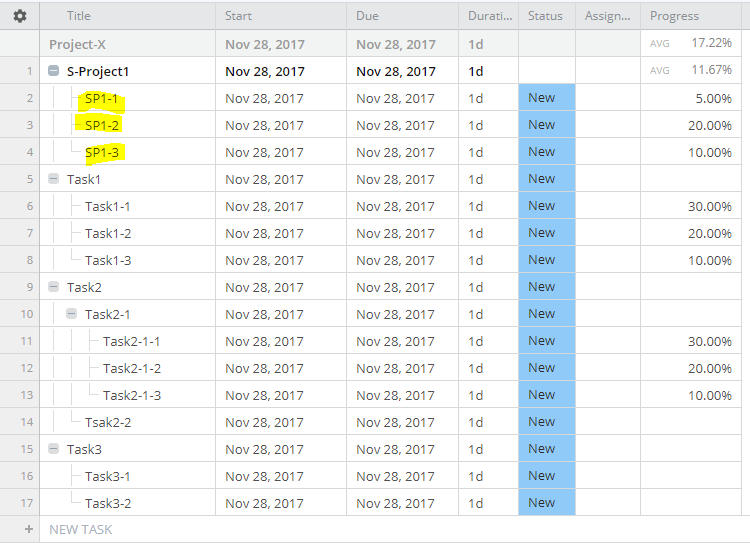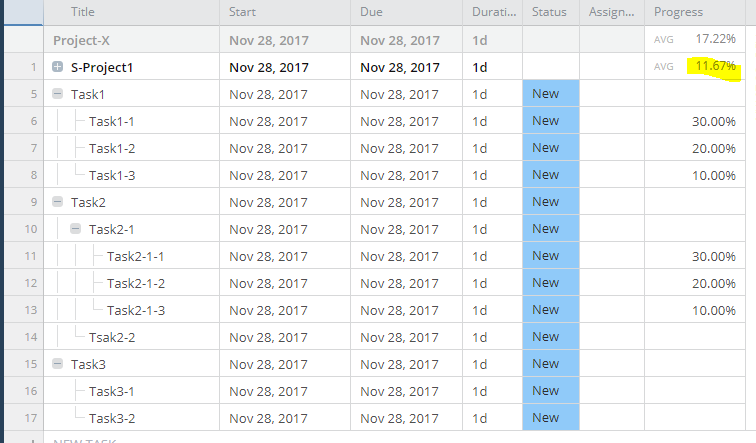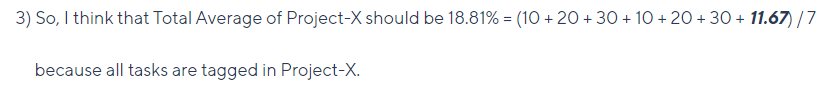# Formula of Average of Custom field

I guess current calculation formula of average may not be appropriate.
Please see my sample of the below.
It is currently calculated as 17.22% = (10 + 20 + 30 + 10 + 20 + 30 + 10 + 20 + 5) / 9.
But I guess it should be 18.81% = (10 + 20 + 30 + 10 + 20 + 30 + 11.67) / 7, if Wrike can calculate sub-total for Sub-Project.
Because the result of calculation seems incorrect if user collapses Sub-Project like below.Akira IT manager

Upvote 0
👍 Spot On 💡 Innovative Approach 💪 Stellar Advice ✅ Solved 🪄 Remove Kudos
4 commentairesStephen

Hi Akira, thanks sharing your feedback in such detail. It really helps us understand your set up 👍

By looking at the screenshot it seems like only subtasks from the Task 1 are being calculated in the field you've highlighted. This is likely due to subtasks from the other Tasks (2, 3) have not been tagged in the parent Project/Folder. By default subtasks only have Ghost Tags that provide, at a glance information, about the subtask's parent, but you need to add the Folder to the subtasks so they 'live' in that Folder.

Can you try adding the subtasks to the parent Folder and see if this helps update the calculations?

Any questions, please let me know!
0
👍 Spot On 💡 Innovative Approach 💪 Stellar Advice ✅ Solved 🪄 Remove Kudos

Hi Stephen,

I guess you might not understand my request correctly.

I was talking about Project-X as Parent Project or Folder.

And I changed Task Names as below because I used same task names in different Parent tasks.1) The total average of Parent project, S-Project1, is correct. (5+20+10=35/3=11.67%)

2) The total average of Task1 & Task2 are not calculated because both are "Task". ( It is not "Project".)

*** It is the best way that the total average is calculated even if the parent is "Task", but I understood it is

the current specification that the total average is not calculated if the parent is "Task".

3) So, I think that Total Average of Project-X should be 18.81% = (10 + 20 + 30 + 10 + 20 + 30 + 11.67) / 7

because all tasks are tagged in Project-X.

Because the result of calculation seems incorrect if user collapses Sub-Project like below.Could you understand the meaning of my request?

Akira IT manager

0
👍 Spot On 💡 Innovative Approach 💪 Stellar Advice ✅ Solved 🪄 Remove Kudos

I'm a few years late Akira Kuragami, but this is a mathematical problem, not a Wrike issue.

You need to add up each item (there are 9 in your example) individually, and take the average of the total. To use your example,If you were to take (10 + 20 + 30 + AVERAGE(10 + 20 + 30) + 11.67) / 5 = 16.43%

The examples can get more ridiculous, where you have 1 task at 100 % completion, and 99 tasks at 1 %

(1 + 1 + 1 + ... + 1 + 100)/100 = 50.5%

(AVERAGE(1 + 1 + 1 + ... + 1 ) + 100) / 2 = 1.99%

So, if you're looking for the average within the project, you assume that each task is equally important, and count them individually. It is incorrect to add some together before averaging. That's not an average.

But, if you say S-Project1 is more important than your individual tasks, then what you're asking for is a weighted average, with the form:

Per Example;  (10*w1 + 20*w2 + 30*w3 + 10*w4 + 20*w5 + 30*w6 + 5*w7 + 20*w8 + 10*w9) / 9 = 18.81%

Assume: w(1,6) = 1

w(7,9) =  1.408

I haven't found a way to run a weighted average properly in Wrike, because any 'weight' term I generate is averaged, denying its original purpose.

Hope this helps

0
👍 Spot On 💡 Innovative Approach 💪 Stellar Advice ✅ Solved 🪄 Remove Kudos

Hi @Tyler-Green, thanks for sharing it! Indeed, this calculation is correct.
As for the weighted average though, may I please ask you to elaborate a little on your use case?

Darina Community Team at Wrike Wrike Product Manager En savoir plus sur les fonctionalités et les meilleures pratiques grace à nos Webinaires online

0
👍 Spot On 💡 Innovative Approach 💪 Stellar Advice ✅ Solved 🪄 Remove Kudos

Folllowing List for Post: Formula of Average of Custom field
[this list is visible for admins and agents only]

Haut de la page